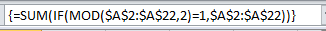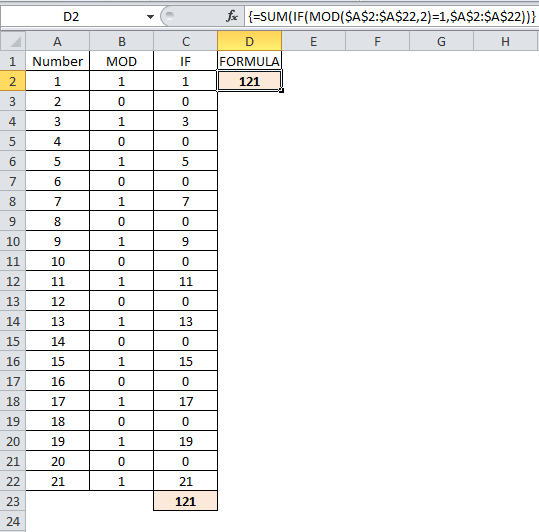# Sum Odd or Even Numbers only in ExcelPardon the pun, but it may seem like an ODD request to want to know how to sum odd or even numbers only in Excel, but it is still a question asked.

Since being asked for help on this, I have started using this formula myself recently where I need to sum odd numbers and then sum even numbers in Excel separately (I won’t get into the boring nitty gritty), but trust me when I say it has proven useful.

To sum odd or even numbers only is done using SUM, IF, MOD and array formula – if you’re put off by this, don’t worry, once explained I hope it makes more sense and is less daunting.

The image above shows the final formula.  The next few sections are going to talk through the logic behind the formula and how I came up with it.  You can skip to the end if you are comfortable with the next steps.This list of numbers will be the basis of what we are about to do.

## MOD

The MOD function “Returns the remainder after a number is divided by a divisor”.

This is our starting point, we know that an odd number when divided by 2 leaves a remainder of 1 (7/2 = 3 with remainder 1).

An even number divided by 2 leaves no remainder (6/2 = 3 with no remainder).

This is how we will distinguish odd from even numbers.

The MOD formula is made up of 2 parameters, the number and the divisor.

The number will be the number in column A and the divisor will be 2.

The formula in cell B2 is =MOD(A2,2).As you can see, all the odd numbers have a remainder of 1 and all the even numbers have a remainder of 0.

## IF

In column C I have entered an IF formula.

If the value in column B (the remainder) is 1, then give the value in column A, or else leave 0.

This then lists all the odd numbers in column C and has 0 in place of the even numbers.

The IF statement is =IF(B2=1,A2,0).In cell C21, there is a simple SUM formula adding up the value of all the odd numbers.

## Sum odd or even numbers in Excel

All of the above might seem a bit longwinded, what we are going to do now is create one array formula that does all of the above, but using the same logic.Let’s breakdown the formula and how it is typed.

You can ignore the {} curly braces for now and I will come back to them after the content between them.

• =SUM( – Obviously, this is going to be a SUM formula and it will SUM whatever i defined between the brackets.
• IF(MOD(\$A\$2:\$A\$22,2)=1, – The logical statement in our IF function is checking if the numbers between A2 and A22 divided by 2, leaves a remainder of 1.  Note the \$ signs in the cell range.  Normally this type of IF function would not work, but this will because we are going to make it an array formula later.
• \$A\$2:\$A\$22)) – The value if true in our IF function will be the values in column A.  If there is a remainder of 1 from our MOD function, then we are going to sum those values here.  There is no value if false parameter in this formula, we are only working on those values that are true.
• {} – The formula as it is will not work.  We need to make it an array formula, to do this, when you key the rest of the formula and while still editing the formula press Ctrl, Shift and Enter/Return together.  Excel makes this an array formula and adds the curly braces {} to the start and end of the formula.To sum even numbers in Excel, change the logical value of your IF statement to check if the MOD function is equal to zero.

There is a bit more on arrays in this formula on VLookUp with Multiple Values.

This idea can be used to SUM or carry out other functions dependent on a certain criteria.

Play around with it yourself and see what you can come up with, or where you might find it useful.